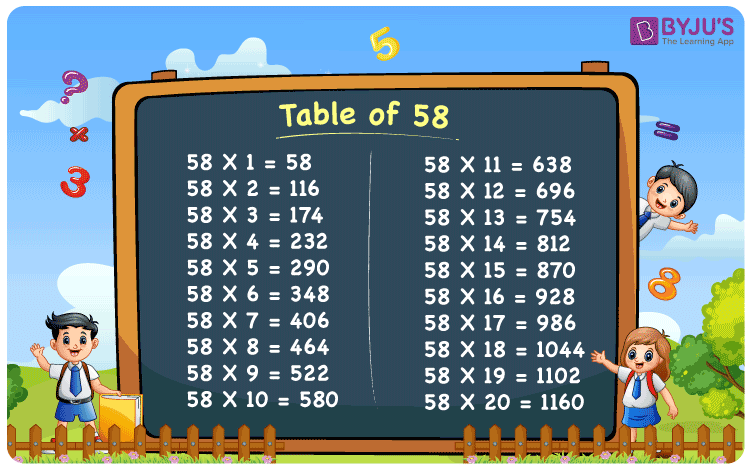# Table of 58

The table of 58 is given here to help the students quickly learn the 58 times table. The multiplication table of 58 is a slightly difficult Maths table compared to the single-digit numbers tables. However, we have provided various writing methods for the 58 times table to make the students understand easily. Also, download the PDF of Maths table 58 to learn anytime, whenever required, without browsing online.

## Table 58 Chart## What is the 58 times table?

Maths tables can be formed using different arithmetic operations such as addition and multiplication. Here, you will learn how to write the table of 58 using multiplication and repeated addition.

 Using multiplication Using repeated addition 58 × 1 = 58 58 58 × 2 = 116 58 + 58 = 116 58 × 3 = 174 58 + 58 + 58 = 174 58 × 4 = 232 58 + 58 + 58 + 58 = 232 58 × 5 = 290 58 + 58 + 58 + 58 + 58 = 290 58 × 6 = 348 58 + 58 + 58 + 58 + 58 + 58 = 348 58 × 7 = 406 58 + 58 + 58 + 58 + 58 + 58 + 58 = 406 58 × 8 = 464 58 + 58 + 58 + 58 + 58 + 58 + 58 + 58 = 464 58 × 9 = 522 58 + 58 + 58 + 58 + 58 + 58 + 58 + 58 + 58 = 522 58 × 10 = 580 58 + 58 + 58 + 58 + 58 + 58 + 58 + 58 + 58 + 58 = 580

## Multiplication Table of 58

The below table represents the multiplication table of 58 up to 20. The results of the following table are also called the first 20 multiples of 58.

 58 × 1 = 58 58 × 2 = 116 58 × 3 = 174 58 × 4 = 232 58 × 5 = 290 58 × 6 = 348 58 × 7 = 406 58 × 8 = 464 58 × 9 = 522 58 × 10 = 580 58 × 11 = 638 58 × 12 = 696 58 × 13 = 754 58 × 14 = 812 58 × 15 = 870 58 × 16 = 928 58 × 17 = 986 58 × 18 = 1044 58 × 19 = 1102 58 × 20 = 1160

## Examples on the Table of 58

Example 1:

Compute: 58 times 9 minus 70

Solution:

58 times 9 minus 70

= 58 × 9 – 70

Using the multiplication table of 58, 58 × 9 = 522.

= 522 – 70

= 452

Example 2:

Arya saves 15 rupees per day. What will be his savings for 58 days?

Solution:

Given that Arya saves Rs. 15 per day.

Total savings by Arya for 58 days = ?

Using the table of 58, 58 × 15 = 870.

Therefore, Arya will save Rs. 870 in 58 days.

## Frequently Asked Questions on Table of 51

### What is the table of 58?

The table of 58 can be formed using multiplication or addition. The multiplication table of 58 contains the multiples of 58. Below is the table of 58 up to 10.
58 × 1 = 58
58 × 2 = 116
58 × 3 = 174
58 × 4 = 232
58 × 5 = 290
58 × 6 = 348
58 × 7 = 406
58 × 8 = 464
58 × 9 = 522
58 × 10 = 580

### In which table does 58 come?

58 comes in two tables, 2 and 29.
2 × 29 = 58
29 × 2 = 58

### How to read 58 times table?

58 times table can be read as: Fifty-eight times one is Fifty-eight, Fifty-eight times two is One hundred and sixteen, Fifty-eight times three is One hundred and seventy-four, Fifty-eight times four is Two hundred and thirty-two, Fifty-eight times five is Two hundred and ninety, Fifty-eight times six is Three hundred and forty-eight, Fifty-eight times seven is four hundred and six, Fifty-eight times eight is Four hundred and sixty-four, Fifty-eight times nine is five hundred and twenty-two, Fifty-eight times ten is Five hundred and eighty, and so on.

### What is the result of 58 times 7?

58 times 7 = 58 × 7 = 406
Therefore, the result of 58 times 7 is 406.